Next: 4.4.2 Vortex Lattice in Up: 4.4 Field Distribution of Previous: 4.4 Field Distribution of

## 4.4.1 Vortex Lattice in a s-Wave Superconductor

The vortex core in a clean s-wave superconductor was first studied by Caroli, de Gennes and Matricon . Using a Bogoliubov formalism these authors calculated the low-energy spectrum of quasiparticle bound states in an isolated vortex core in which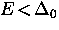,whereis the bulk energy gap in zero magnetic field. They determined that the vortex core radius r0 (i.e. where the order parameter changes appreciably) was on the order of the coherence length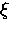. The model is conceptually the same as the quantum mechanical picture of a particle in a cylindrical potential well of radius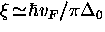and depth.The eigenvalues of the low-lying quasiparticle states may be written as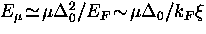, where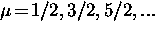, are the angular momentum quantum numbers and EF is the Fermi energy.Bardeen et al.  later extended the calculations of Caroli et al. to determine the higher energy scattering states with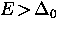and the effects of the magnetic field on the energy of the bound states in the vortex core. Neumann and Tewordt  determined the vortex structure near Tc by numerically solving the GL equations while various other authors obtained vortex-lattice solutions of the Gor'kov microscopic equations [128,129] near Hc2 using a variety of approximations [130,131,132,133,134].

To determine the vortex structure for arbitrary temperature, magnetic field and impurity concentrations, numerous efforts were made to numerically solve Eilenberger's equations , which are a reformulation of the microscopic Gor'kov theory. In the dirty limit the transport-like Eilenberger equations reduce to the simpler diffusion-like Usadel equations [136,137]. Using a circular-cell approximation, Kramer et al.  determined the vortex structure in the dirty limit by numerically solving the Usadel equations near Hc1. They found that the size of the vortex core shrinks with decreasing T at low temperatures, but that the effect is much weaker than expected in the clean limit. Numerical solutions of the Eilenberger equations for nearly isolated vortices in the clean limit were later obtained [139,140], again using a circular-cell approximation. In the clean limit the size of the vortex core region was found to shrink more drastically and the field at the center of the core increased with decreasing temperature. In particular, for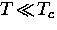the order parameter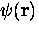and the supercurrent density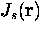are predicted to increase from the center of an isolated vortex core over a length scalewhich has a temperature dependence given by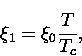(9)
where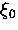is the coherence length defined in BCS theory. This prediction is commonly referred to as the Kramer-Pesch effect''. The predicted temperature dependence of the vortex core size is related to thermally activated quasiparticle excitations which populate the higher energy bound states. At high temperatures the bound states with energies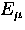are densely packed within the low-energy region of the vortex core (i.e.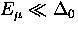). Upon reducing the temperature, the bound state energiesincrease so that there are fewer bound states in the vortex core region. The mimimum size of the vortex core is obtained when only the lowest bound state is populated . The case of arbitrary impurity concentration was treated in the context of the Eilenberger equations using a circular-cell approximation by Rammer et al.  and by Klein , who rigorously solved the Eilenberger equations numerically for a hexagonal vortex lattice without making any approximations. Impurities reduce the maximum field in the vortex core. Near Tc the effects of impurities on the magnetic field distribution are negligible.

More recently, Hayashi et al.  have investigated the quantum regime of a single vortex in a clean s-wave superconductor, by self-consistently solving the Bogoliubov-de-Gennes (BdG) equations. The temperature dependence of the vortex-core radius is found to essentially agree with the Kramer-Pesch effect except at temperatures below the so-called quantum limit''. In this limit the temperature is low enough that there is no thermal smearing of the discrete bound quasiparticle states in the vortex cores (i.e.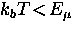). Acccording to Hayashi et al., the quantum limit is reached below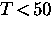mK in NbSe2. In this temperature region the shrinkage of the vortex cores must saturate. Unfortunately, experiments thus far have not probed this low-temperature regime.

Scanning tunneling microscopy (STM) experiments on the layered hexagonal conventional type-II superconductor NbSe2 by Hess et al.  confirmed the existence of localized states in the cores. In the vicinity of a single vortex they measured the differential conductance dI/dV, which is proportional to the local density of states (LDOS). Well away from the vortex center, the dI/dV vs. V scan resembled the standard BCS density of states for zero magnetic field. In the vortex core region, however, instead of the constant LDOS expected for the normal state, they observed a pronounced peak in the differential conductance centered at zero bias voltage. The peak has been interpreted as being due to the bound states localized inside the vortex core. Theoretical efforts [145,146,147,149,150] shortly followed which focused on calculating the LDOS observed in this now famous experiment and subsequent experiments on NbSe2 by Hess et al. [151,152,153]. In a conventional s-wave superconductor with an isotropic energy gap, the LDOS has circular symmetry around an isolated vortex core. Hess et al. observed that the LDOS has a sixfold star shape around a vortex in NbSe2 . Furthermore, the orientation of the star was found to depend on the quasiparticle energy and at intermediate bias voltages the rays split . The origin of the sixfold symmetry in the LDOS has been explained in terms of vortex-vortex interactions , gap anisotropy , the anisotropic Fermi surface , and combinations of these effects . The magnetic field distribution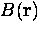in the vortex core region will depend on which of these interpretations is correct. For instance, if vortex-vortex interactions are the major source then the field distributionwill be nearly circularly symmetric in the vortex core region and will progress to a definite sixfold symmetry farther away from the vortex center (as shown in Ref. ). The circular symmetry will extend further out from the vortex center as the magnetic field is weakened. On the other hand, if the sixfold symmetry observed in the STM experiments is due to an anisotropic s-wave energy gap, the sixfold symmetry inwill be more prominent in the vortex core region. Even in this latter case, however, the field distribution in the vortex core region can be reasonably approximated by circular symmetry.

The GL theory has the spatial dependence of the order parameter built in and thus provides a natural description of the magnetic field in the vortex-core region. Abrikosov  predicted the vortex state from his famous periodic solution of the GL equations near Hc2. He also provided an approximate analytical solution of the GL equations for an isolated vortex near Hc1. For intermediate fields, the GL equations must be solved numerically. The magnetic field distribution obtained from the exact numerical solutions of the GL equations coincides with that from the modified London model at low fields and arbitrary. J.R. Clem  proposed a variational model to solve the GL equations based upon a trial function for the order parameter: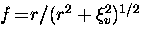, where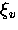is a variational core radius parameter. This model solves the GL equations approximately at low magnetic fields (i.e. isolated vortices) yielding an analytical expression for the magnetic field distribution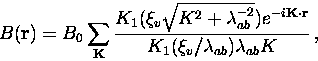(10)
where K1 (x) is a modified Bessel function. For extreme type-II superconductors (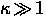),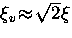, whereis the GL coherence length.

Hao et al.  extended the Clem model to larger magnetic fields through the linear superposition of the field profiles of the individual vortices. This included multiplying the trial function for the order parameter by a second variational parameter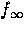to take into account the depression of the order parameter due to the overlapping of vortices. In particular,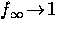as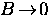(i.e. the Clem limit) and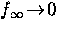as B approaches the upper critical field. Yaouanc et al.  recently simplified Hao's analytical model exclusively to the case of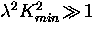, where Kmin is the smallest non-zero reciprocal lattice vector of the vortex lattice. This condition is satisfied even at low fields for largesuperconductors like the high-Tc compounds. The result is that the local field at any point in the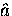-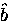plane due to an applied field along the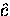-axis is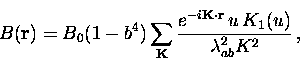(11)
where K1 (u) is a modified Bessel function and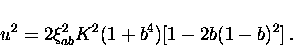(12)
The Bessel function has the asymptotic limits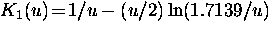for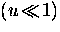and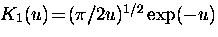for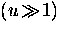.Yaouanc et al.  argued that there is no general theory for the field distribution valid at arbitrary temperature and that thederived from GL theory should be applicable down to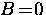. This seems unliklely since the symmetry of the LDOS around a vortex core depends on the energy E of the quasiparticle bound states relative to the temperature dependent energy gap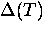.Nevertheless, Brandt  has recently developed an iteration method for solving the GL equations, to compute the field profilefor a vortex lattice of arbitrary symmetry at any value of magnetic field. Equation (4.13) is found to agree extremely well with these exact numerical solutions of the GL equations at low reduced fields b .

To employ Eq. (4.10) or Eq. (4.13) one must assume an appropriate geometry for the vortex lattice. Theoretically, the equilibrium structure of the vortex lattice can be found by minimizing the Gibbs free energy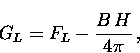(13)
where the London free energy per unit volume associated with the vortices is(14)
where A is the area of the sample. The vortex-lattice geometry which minimizes the free energy for a conventional s-wave superconductor is a triangular lattice [162,163,164].

Ideally, the vortex structure is determined by experiment. This is possible in superconductors which contain few foreign contaminants or structural defects. For instance, STM [144,153] and small angle neutron scattering (SANS)  measurements on the anisotropic conventional type-II superconductor NbSe2 show a perfect triangular lattice with long-range order, when the magnetic field is applied parallel to the-axis.Next: 4.4.2 Vortex Lattice in Up: 4.4 Field Distribution of Previous: 4.4 Field Distribution of